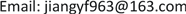1. 研究背景

2. 定价模型

2.1. 价格影响因素

2.1.1. 任务密集程度

2.1.2. 会员密集程度

d A B = π R 180 ( θ A − θ B ) 2 + ( φ A − φ B ) 2 (1)

1) 任务分布密集程度与定价水平负相关；

2) 会员分布密集程度与定价水平负相关；

3) 任务密集区周边，会员分布较稀疏地区任务定价较高。

Basic information of tasks and members in A, B,

2.1.3. 任务完成率

2.2. 定价模型

2.2.1. 区域定价方程

1) 人均任务量： ρ = T i M i ；2) 信誉程度： C i = ∑ i c i j M i ；3) 限额水平： L i j = ∑ j l i j M i

P = α M i + β (2)

F i = ϕ M i + φ l i j + δ r i (3)

p i = 1 1 + e − f i (4)

2.2.2. 任务打包

1) 层次聚类

2) 打包后的任务定价

3. 模型评价

4. 总结

The comparison before and after optimized price
A区域B区域C区域